# Introduction

`vtree` is a flexible tool for generating variable trees — diagrams that display information about nested subsets of a data frame. Given simple specifications, `vtree` produces these diagrams and automatically labels them with counts, percentages, and other summaries. With `vtree`, you can:

• explore a data set interactively, and

• produce customized figures for reports and publications.

## Two examples

Subsets play an important role in almost any data analysis. Imagine a data set of countries, with variables named `population`, `continent`, and `landlocked`. We might wish to examine subsets of the data set based on the `continent` variable. Within each of these subsets, we might wish to examine nested subsets based on the `population` variable, for example, countries with populations under 30 million and over 30 million. We might continue to a third level of nesting based on the `landlocked` variable. `vtree` provides a general solution to the problem of calculating nested subsets and displaying information about them. Nested subsets help us to answer questions like the following: Among African countries with a population over 30 million, what percentage are landlocked?

The variable tree below answers this question: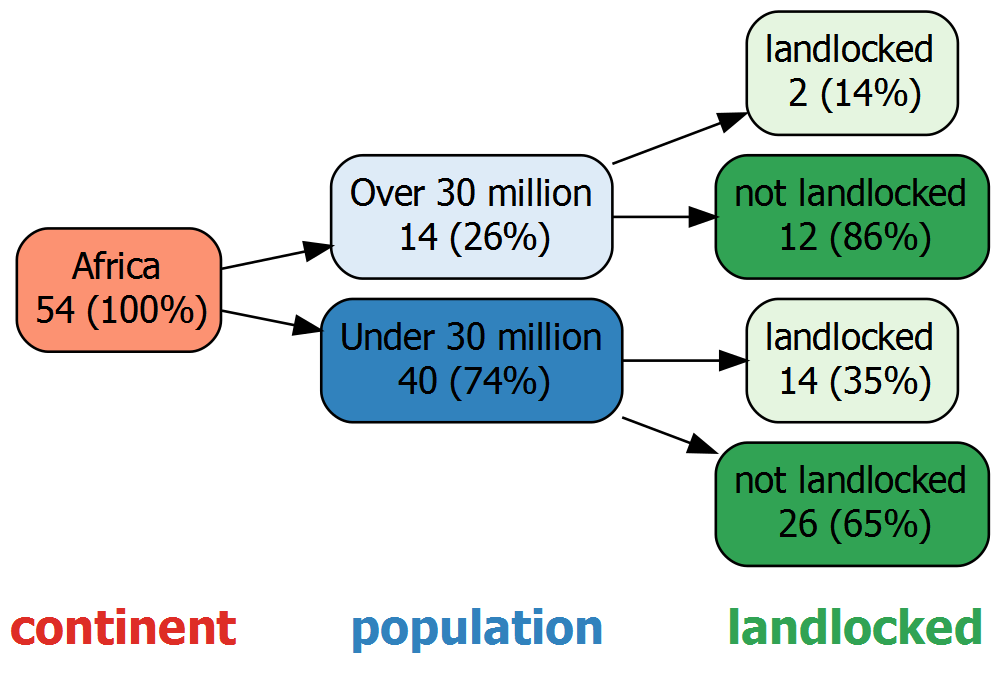Even in simple situations like this, it can be a chore to keep track of nested subsets and to calculate percentages. But it’s often even more tedious—and there are two reasons why. First, the presence of missing values makes it harder to determine denominators. Second, as the number of variables increases, the number of nested subsets grows rapidly. In spite of these difficulties, people often calculate nested subsets by hand (along with percentages and other summaries). Not only is this tiresome work, it is extremely error prone.

Nested subsets arise in all kinds of situations. Consider, for example, flow diagrams for clinical studies, such as the following rudimentary CONSORT diagram, which is also a variable tree.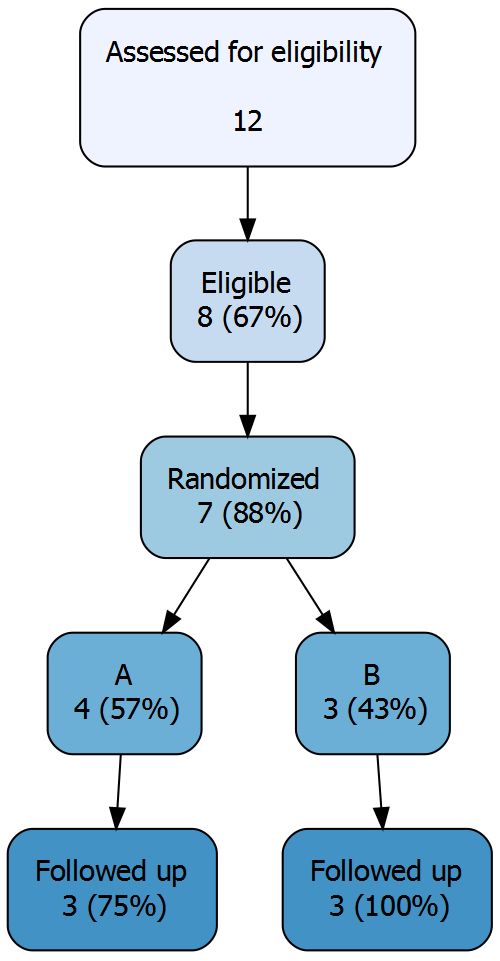Because manual calculation and transcription are error-prone, mistakes in published flow diagrams are all too common. And although the errors that make it to publication are often small, they can sometimes be disastrous.

Note that at the end of this vignette, there is a collection of examples using R datasets that you can try.

## Basic features of a variable tree

The examples that follow use a data set called `FakeData` which represents 46 fictitious patients. The variable tree below depicts subsets defined by `Sex` (M or F) nested within subsets defined by disease `Severity` (Mild, Moderate, Severe, or NA). Although this example—and many subsequent ones—use just two variables, variable trees are especially useful with three or more variables.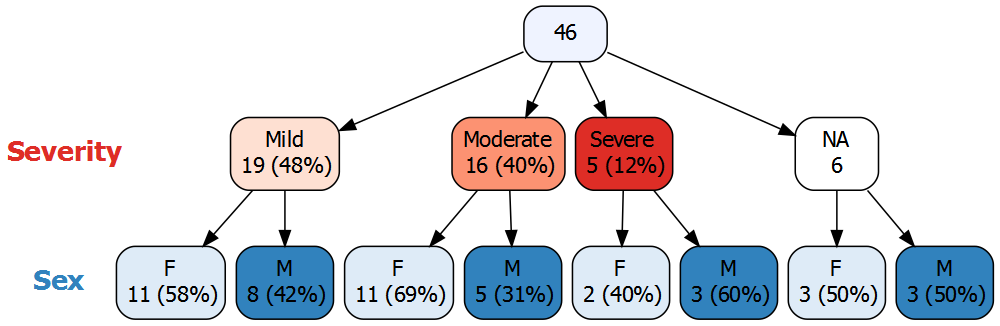A variable tree consists of nodes connected by arrows. At the top of the diagram above, the root node of the tree contains all 46 patients. The rest of the nodes are arranged in successive levels, where each level corresponds to a variable. Note that this highlights one difference between variable trees and some other kinds of trees: at each level of a variable tree, regardless of the branch, the nodes represent values of the same variable. (Decision trees, in contrast, can have splits on different variables at the same level.)

Continuing with the variable tree above, the nodes immediately below the root represent values of `Severity` and are referred to as the children of the root node. In this case, `Severity` was missing (NA) for 6 patients, and there is a node for these patients. Inside each of the nodes, the number of patients is displayed and—except for in the missing value node—the corresponding percentage is also shown. Note that, by default, `vtree` displays “valid” percentages, i.e. the denominator used to calculate the percentage is the total number of non-missing values, 40.

The final level of the tree corresponds to values of `Sex`. These nodes represent males and females within subsets defined by each value of `Severity`. In each of these nodes the percentage is calculated in terms of the number of patients in its parent node.

Like any node, a missing-value node can have children. For example, of the 6 patients for whom `Severity` is missing, 3 are female and 3 are male. By default, `vtree` displays the full missing-value structure of the specified variables.

Also by default, `vtree` automatically assigns a color palette to each variable. `Severity` has been assigned red hues (lightest for Mild, darkest for Severe), while `Sex` has been assigned blue hues (light blue for females, dark blue for males). The node representing missing values of `Severity` is colored white to draw attention to it.

## Variable trees compared to contingency tables

A tree with two variables is similar to a two-way contingency table. In the example above, `Sex` is shown within levels of `Severity`. This corresponds to the following contingency table, where the percentages within each column add to 100%. These are called column percentages.

Mild Moderate Severe NA
F 11 (58%) 11 (69%) 2 (40%) 3 (50%)
M 8 (42%) 5 (31%) 3 (60%) 3 (50%)

Likewise, a tree with `Severity` shown within levels of `Sex` corresponds to a contingency table with row percentages.

The contingency table above is more compact than the corresponding variable tree, but some people may find the variable tree easier to interpret. When three of more variables are of interest, multi-way contingency tables are often used. These are typically displayed using several two-way tables. In this situation, variable trees are generally easier to interpret.

It is also worth noting that contingency tables are not always more compact than variable trees. When most cells of a large contingency table are empty (in which case the table is said to be sparse), the corresponding variable tree may be more compact since empty-nodes are not shown.

## Features of vtree

vtree is designed to be quick and easy to use, so that it is convenient for data exploration, but also flexible enough that it can be used to prepare publication-ready figures. To generate a basic variable tree, it is only necessary to specify a data frame and some variable names. However extra features extend this basic functionality to provide:

In many cases, you may wish to generate several different variable trees to investigate a collection of variables in a data frame. For example, it is often useful to change the order of variables, prune parts of the tree, etc.

## Technical overview

vtree is built on open-source software: in particular Richard Iannone’s DiagrammeR package, which provides an interface to the Graphviz software using the htmlwidgets framework. Additionally, vtree makes use of the Shiny package, and the svg-pan-zoom JavaScript library.

A formal description of variable trees follows.

The root node of the variable tree represents the entire data frame. The root node has a child for each observed value of the first variable that was specified. Each of these child nodes represents a subset of the data frame with a specific value of the variable, and is labeled with the number of observations in the subset and the corresponding percentage of the number of observations in the entire data frame. The nth level below the root of the variable tree corresponds to the nth variable specified. Apart from the root node, each node in the variable tree represents the subset of its parent defined by a specific observed value of the variable at that level of the tree, and is labeled with the number of observations in that subset and the corresponding percentage of the number of observations in its parent node.

Note that a node always represents at least one observation. And unlike a contingency table, which can have empty cells, a variable tree has no empty nodes.

# The `vtree` function

Consider a data frame named `df`, which includes discrete variables `v1` and `v2`. In this case, a variable tree can be displayed using the following command:

``vtree(df,"v1 v2")``

For additional details about how variables can be specified, see the section on specification of variables below. Note that if `vtree` is called without a list of variables, it uses as many discrete variables from the data frame as possible, starting with the first column.

``````NOTES:
1. Discrete variables are operationally defined here as variables with at most 4 distinct values.
2. Variables are included until the maximum number of nodes exceeds the maxNodes parameter value.``````

Numerous additional parameters can be supplied. For example, by default `vtree` produces a horizontal tree (that is, a tree that grows from left to right). To generate a vertical tree, specify `horiz=FALSE`.

## Mini tutorial

To display a variable tree for a single variable, say `Severity`, use the following command:

``vtree(FakeData,"Severity")``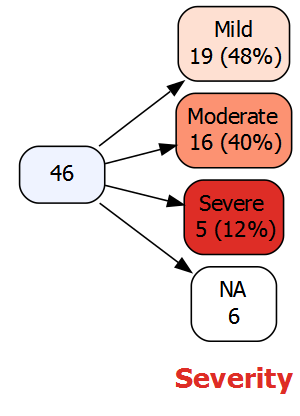Next, consider a vertical variable tree with two variables, `Severity` and `Sex`. A less colorful display with more spacing can be requested by specifying `plain=TRUE`:

``vtree(FakeData,"Severity Sex",horiz=FALSE,plain=TRUE)``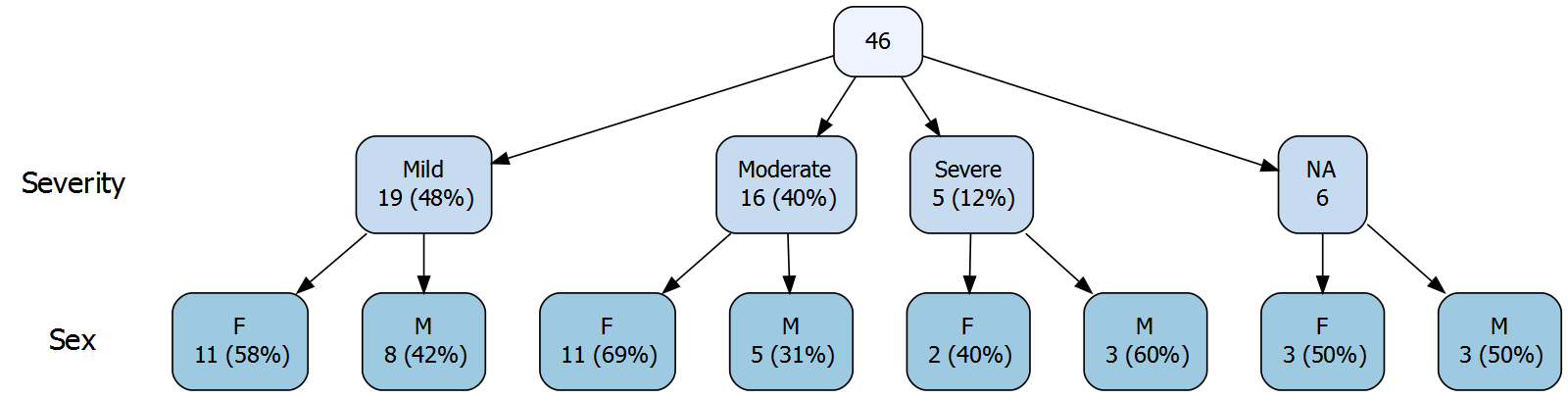### Percentages

By default, “valid percentages” are shown, i.e. the denominator is the total number of non-missing values. In the case of `Severity`, there are 6 missing values, so the denominator is 46 - 6 , or 40. There are 19 Mild cases, and 19/40 = 0.475 so the percentage shown is 48%. No percentage is shown in the NA node since missing values are not included in the denominator.

If you prefer that the denominator represent the complete set of observations (including any missing values), specify `vp=FALSE`. With this setting, a percentage will be shown in each of the nodes, including any NA nodes.

If you don’t wish to see percentages, specify `showpct=FALSE`, and if you don’t wish to see counts, specify `showcount=FALSE`.

### Displaying a legend and hiding node labels

To display a legend, specify `showlegend=TRUE`. Next to each level of the tree, the variable name is displayed together with color discs and the values they correspond to. For each of the values, overall (marginal) counts are shown, together with percentages.

When the legend is shown, the node labels become redundant, since the colors identify the values of the variables (although the labels may aid readability). If you prefer, you can hide the node labels, by specifying `shownodelabels=FALSE`:

``vtree(FakeData,"Severity Sex",showlegend=TRUE,shownodelabels=FALSE)``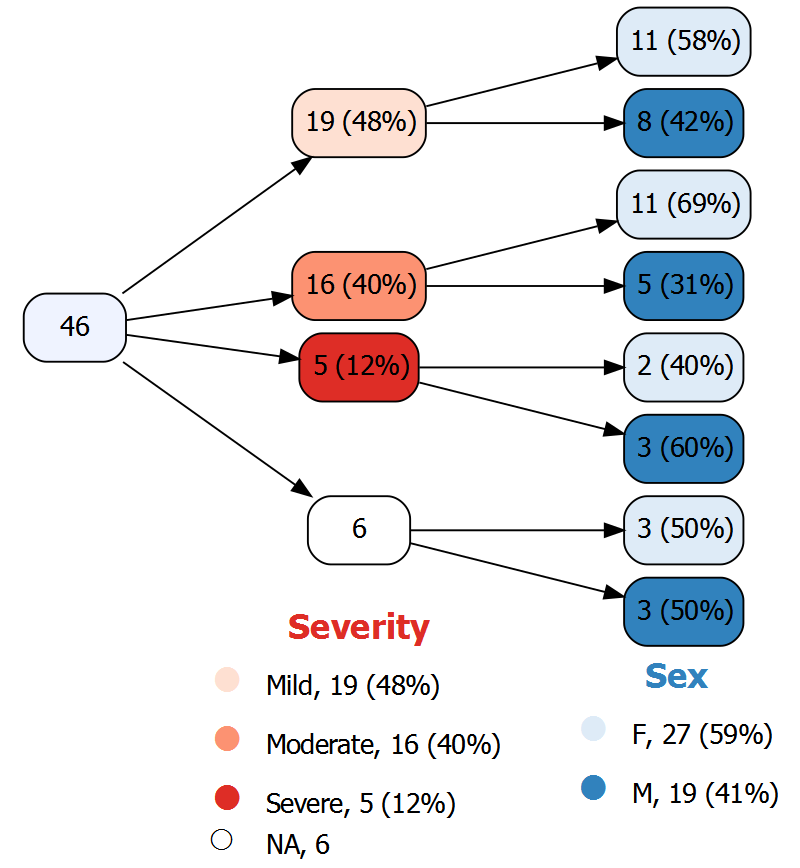The legend shows how colors are assigned to the different values of each variable, and additionally provides marginal (that is, overall) counts and percentages for each variable. Since `Severity` is the first variable in the tree—i.e., it is not nested within another variable— the marginal counts and percentages for `Severity` are identical to those displayed in the nodes. In contrast, for `Sex`, the marginal counts and percentages are different from what is shown in the nodes because the nodes for `Sex` are nested with levels of `Severity`.

(Unfortunately the NA circle in the legend is oddly sized and positioned due to an issue with the corresponding unicode symbols.)

### Putting node labels on the same line as the counts and percentages

When a variable tree is large, it can be difficult to display it in a readable way. One approach that helps is to display the tree horizontally and also to put the node labels on the same line as the counts and percentage by specifying `sameline=TRUE`. For example, the following results in nodes with single-lines labels such as Moderate, 16 (40%), etc.:

``vtree(FakeData,"Severity Sex Viral",sameline=TRUE)``

### Hiding variable names

By default, next to each level of the tree, `vtree` shows the variable name. These can be removed by specifying `showvarnames=FALSE`.

### Text wrapping

By default, `vtree` wraps text onto the next line whenever a space occurs after at least 20 characters. This can be adjusted, for example, to 15 characters, by specifying `splitwidth=15`. To disable line splitting, specify `splitwidth=Inf`. Text wrapping in the legend is controlled independently. To set the splitting in the legend to 8 characters, specify `lsplitwidth=8`. Also note that in the legend, text wrapping can take place not only at spaces, but also at any of the following characters: . - + _ = /

This concludes the mini-tutorial. `vtree` has many more features, described in the following sections.

## Pruning

When a variable tree gets too big, or you are only interested in certain parts of the tree, it may be useful to remove some nodes along with their descendants. This is known as pruning. For convenience, `vtree` provides several different ways to prune a tree, described below.

### The `prune` parameter

Suppose you don’t want the tree to include individuals whose disease is Mild or Moderate. Specifying `prune=list(Severity=c("Mild","Moderate"))` removes those nodes, and all of their descendants:

``vtree(FakeData,"Severity Sex",prune=list(Severity=c("Mild","Moderate")))``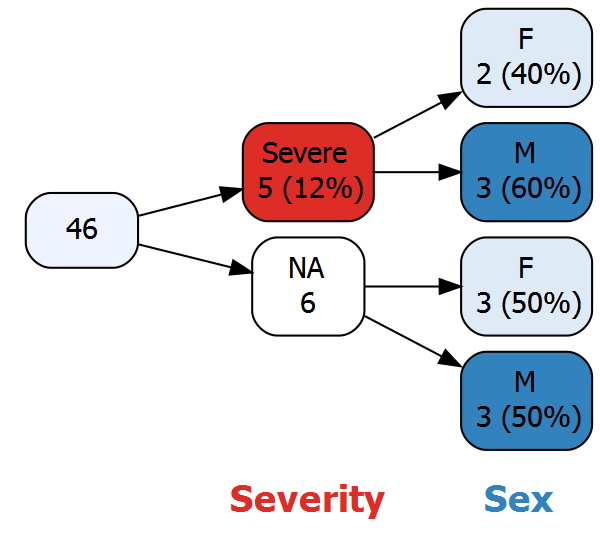In general, the argument of the `prune` parameter is a list with an element named for each variable you wish to prune. In the example above, the list has a single element, named `Severity`. In turn, that element is a vector `c("Mild","Moderate")` indicating the values of `Severity` to prune.

Caution: Once a variable tree has been pruned, it is no longer complete. This can sometimes be confusing since not all observations are represented at certain levels of the tree. It is particularly important to avoid pruning missing value nodes, since this makes it hard to interpret “valid” percentages (i.e. percentages calculated using the number of non-missing observations as denominator).

### The `keep` parameter

Sometimes it is more convenient to specify which nodes should be retained rather than which ones should be discarded. The `keep` parameter is used for this purpose, and can thus be considered the complement of the `prune` parameter.

For example, to retain only the Moderate `Severity` node:

``vtree(FakeData,"Severity",keep=list(Severity="Moderate"))``

#### Missing value nodes and the `keep` parameter

It is important to note how the `keep` parameter functions when missing values are present. Consider a variable tree for the `Severity` variable, shown on the left with so-called “valid” percentages. These are percentages calculated using the number of non-missing observations as denominator (which is the default, specified by `vp=TRUE`). On the right is the same tree, but with percentages calculated using the total number of observations as the denominator.

``````vtree(FakeData,"Severity")
vtree(FakeData,"Severity",vp=FALSE)``````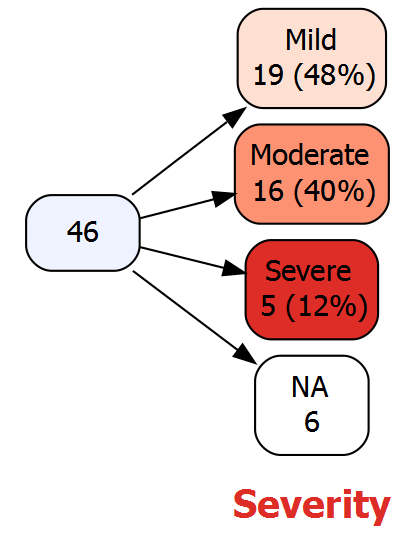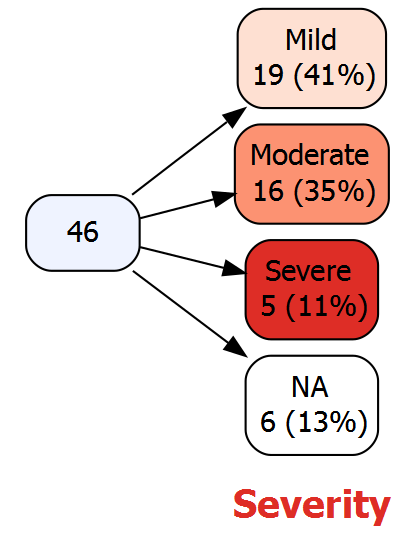Suppose we use `keep` to retain only the Moderate node.

``````vtree(FakeData,"Severity",keep=list(Severity="Moderate"))
vtree(FakeData,"Severity",vp=FALSE,keep=list(Severity="Moderate"))``````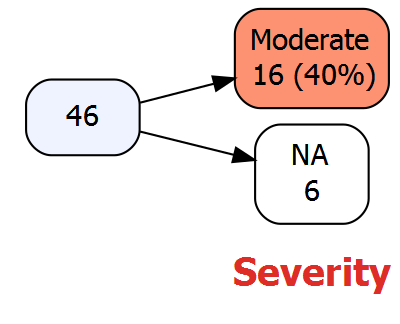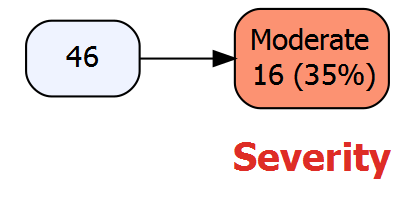Note that in the tree on the left (which uses valid percentages), the NA node is retained. This is done so that the percentage of Moderate cases can be interpreted.. (There are 16 Moderate cases, and a total of 40 non-missing cases, which is 40%.)

On the right, the NA node has been removed, because the denominator (46) doesn’t depend on the number of missing values.

### The `prunebelow` parameter

A disadvantage of the `prune` parameter is that in the resulting tree, the counts shown in child nodes may not add up to the counts shown in the parent node. For example in the variable tree above, of a total of 46 patients, 5 have Severe disease and `Severity` is unknown for 6. One might wonder what happened to the other 35 patients.

A solution to this problem is to retain the specified nodes, but to prune below them (i.e. to prune their descendants). In the present example, this means that the Mild and Moderate nodes will be shown, but not their descendants. The `prunebelow` parameter is used to do this, and its argument has the same form as for the `prune` parameter.

``vtree(FakeData,"Severity Sex",prunebelow=list(Severity=c("Mild","Moderate")))``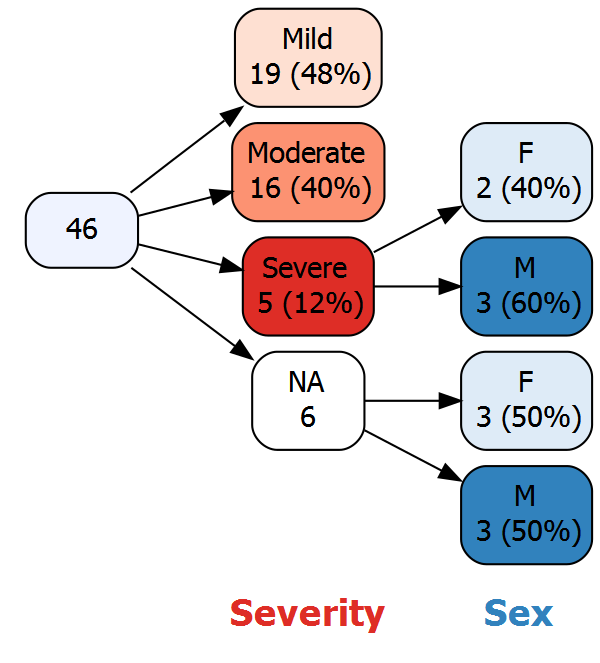### The `follow` parameter

The complement of the `prunebelow` function is the `follow` function. Instead of specifying which nodes should be pruned below, this allows you to specify which nodes should be “followed” (that is, not pruned below).

### The `prunesmaller` parameter

As a variable tree grows it can become difficult to see the forest for the tree. For example, consider the following variable tree:

``vtree(FakeData,"Severity Sex Age Category",sameline=TRUE)``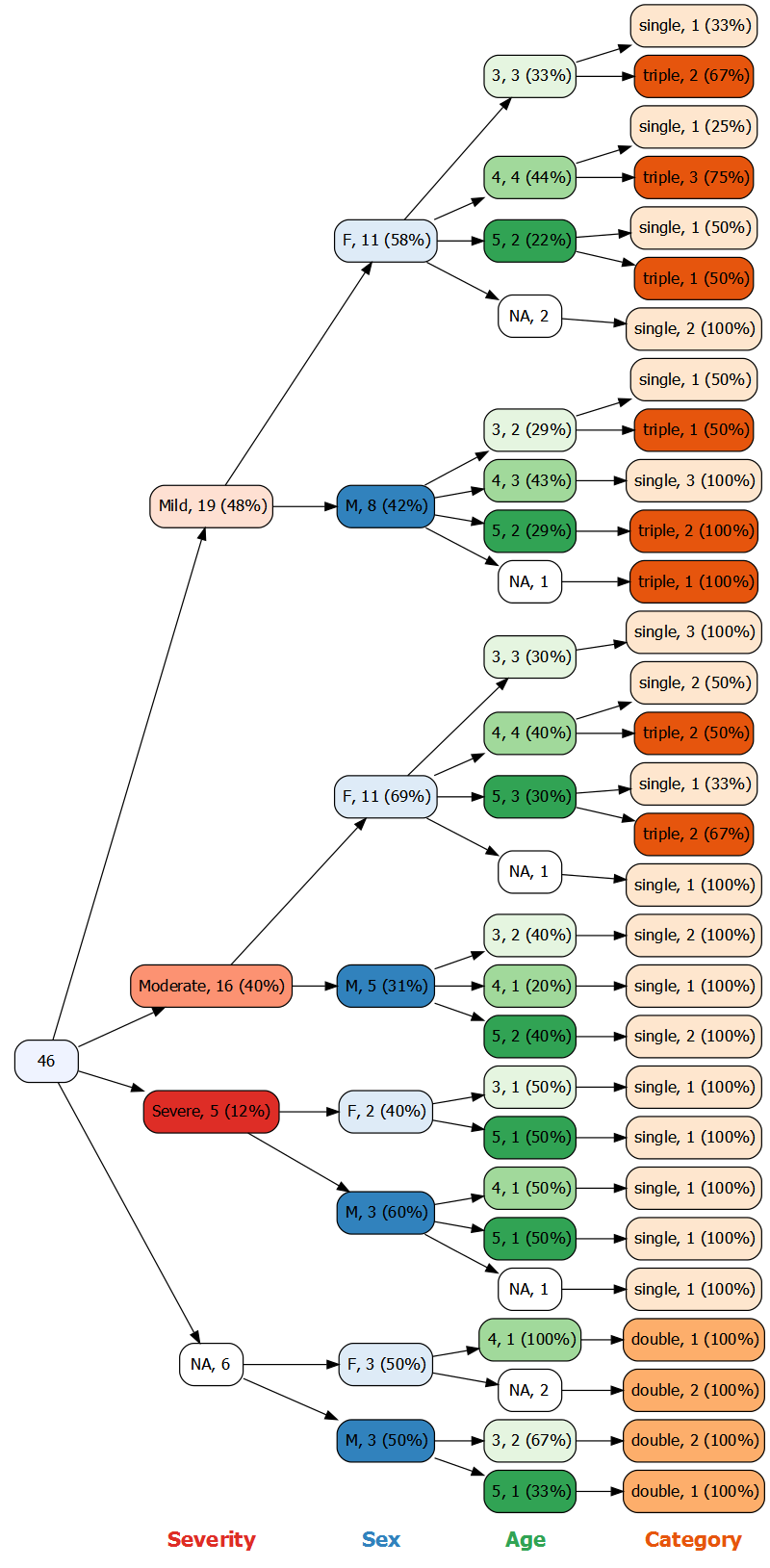One solution is to prune nodes that contain small numbers of observations. For example if you want to only see nodes with at least 3 observations, you can specify `prunesmaller=3`, as in this example:

``vtree(FakeData,"Severity Sex Age Category",sameline=TRUE,prunesmaller=3)``